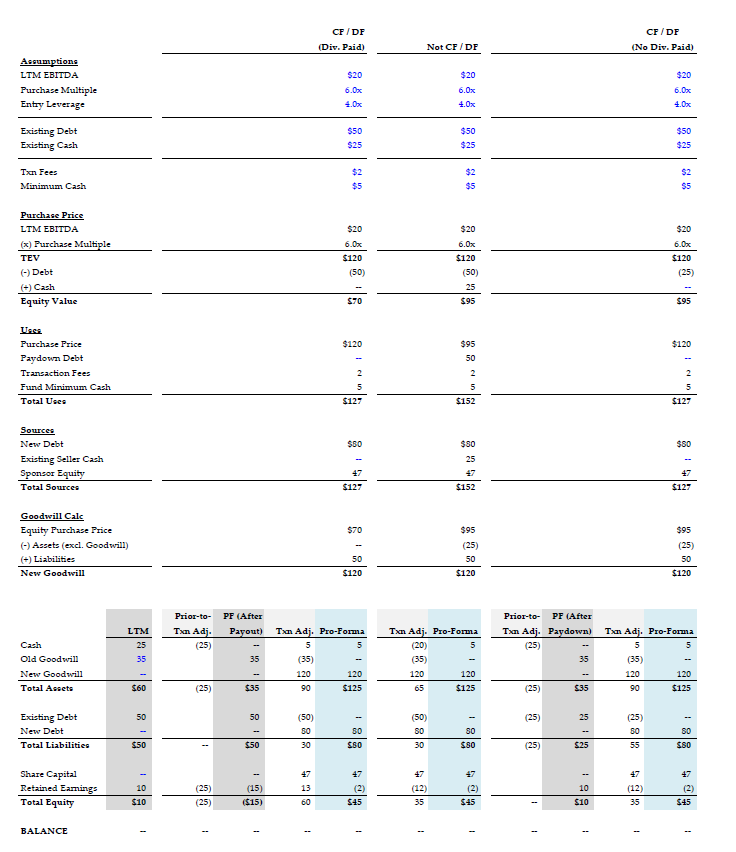Hi all - Been trying to fully understand the sources & uses of CF/DF LBOs vs. Non-CF/DF LBOs. The picture is an example. Am I approaching this the right way? Thanks.

I'll try and tackle this, although there will be some assumptions being made on my end so I apologize if I mess something up.

1. Enterprise to Equity Value walk. EV = Equity Value + Debt - Cash (aka + Net Debt). However, we only care about debt on a cash free basis if we assume the equity shareholder's will be keeping the cash as cash is effectively 0. On a debt free basis enterprise value = transaction value. If we are assuming the debt rolls forward than transaction value = equity value. So rearranging if we assume the equity shareholder's keep the cash (cash free) the equity value should be Equity = EV - Debt or equal to 120 - 50 = 70. If they didn't it would the normal walk of Equity = EV - Net Debt. Most importantly remember that CF / DF or not enterprise value remains the same.

2. For CF / DF your sources and uses are correct (assuming the new \$80 in debt is the new buyers debt). Depending on how the transaction is set up you may further want to break out the purchase price into debt and equity just to convey what is happening with the transaction. I.e. show that the B/S had debt on it before.

3. On the second this will look like a normal transaction. Use cash from the balance sheet to fund the transaction, show pay down debt on the uses of \$50, and then show the equity value as \$95 instead of \$145. Remember net debt is positive here (debt > cash) the only time we see equity > enterprise value is when net debt is negative (like after a VC round when post money > pre money due to the excess cash on the balance sheet).

Hopefully that helps

Hi! Thanks for the catch, had meant to do TEV - debt + cash. Slip-up on my part. Just updated the picture. Can you confirm if this is correct now?

And to clarify, the example is assuming equity holders do not pay themselves a dividend with cash prior to close (Edit: although, in CF/DF, is it just assumed that the seller pays themselves the dividend with all the remaining cash? So by default in this example, equity value should be 70? Or is this something that's more up to discretion even in CF / DF?)

Yes looks correct. Someone please feel free to correct me as well lol.

One comment, someone might ask: well transaction values are different now aren't transaction fees a % of transaction size etc. For your purposes I would just hold steady. The EA in real life might vary though.

On the other question, I've actually seen both ways which is, as you know, generalizations with transactions aren't fun lol.

From the technically correct / interview correct I would say that the best assumption to be made is that, yes, the seller always pays themselves a dividend on a CF / DF transaction before the sale.

Thanks so much! Super helpful given I have a test tomorrow lol.

Just updated the picture again with PF balance sheet. So if I'm understanding correctly, regardless of CF / DF and if cash is paid out beforehand or not, this doesn't impact the LBO since you end up with the same PF balance sheet, sponsor equity, and debt amount right? It's essentially just shifting cash/debt between sources and uses?

Only thing it would change is the implied equity value of the target, so it could affect premiums paid?

Yea I believe you are exactly right.

What this question really is is just the big kid version of what happens to enterprise value if you find \$100 on the sidewalk and put it in your back account lol. Good luck.

Awesome, appreciate it man :) Hopefully this helps out some other monkeys!

hi, thank you for sharing this, am going through the example here but would like to clarify the non-CFDF S&U. Given non-CFDF means that buyer gets to assume existing debt and keep cash (but they'd still have to pay for cash - all seller would want to be compensated for cash) i would have thought that the S&U for non-CFDF to be:

Uses

Purchase equity = 95

Assume existing debt = 50

Transaction fees = 2

Fund min cash = 5

Total uses = 152

Sources

Assume existing debt = 50

Cash on BS (which seller paid for in purchase equity) = 25

New debt = 50

Total sources = 152

The way i'm thinking about it is that non-CFDF means that the seller can assume existing debt hence reducing equity contribution - not sure what i'm missing here? really appreciate any clarification, thank you!

Your S&U is technically correct, although for LBOs in the context of an interview / model test, my impression / experience has been to assume refinancing of the existing debt using new debt.

The key issue in your example is regardless of whether you assume the existing debt or take on new debt, a key limitation in LBOs is your pro forma leverage. You're pro forma debt is \$100mm vs. my \$80mm, which would push the leverage ratio above the limit of 4.0x in the assumptions.

If you stick with the leverage limit and assume a carryover of the existing debt, you would have (a) Assume Existing Debt = 50, (b) Existing Seller Cash = 25, (c) New Debt = 30, (d) Sponsor Equity = 47

So you end up in the same place.

Following

Array

Sorry also edited some wording in my original post - grateful for any views